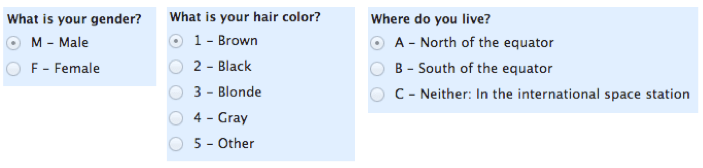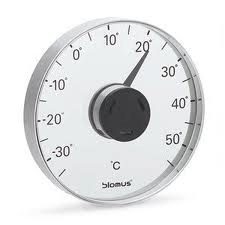# Types of Data & Measurement Scales

## Types of Data & Measurement Scales: Nominal, Ordinal, Interval and Ratio

There are four measurement scales (or types of data): nominal, ordinal, interval and ratio.  These are simply ways to categorize different types of variables.  This topic is usually discussed in the context of academic teaching and less often in the “real world.”  If you are brushing up on this concept for a statistics test, thank a psychologist researcher named Stanley Stevens for coming up with these terms.  These four measurement scales (nominal, ordinal, interval, and ratio) are best understood with example, as you’ll see below.

Nominal
Let’s start with the easiest one to understand.  Nominal scales are used for labeling variables, without any quantitative value.  ”Nominal” scales could simply be called “labels.”  Here are some examples, below.  Notice that all of these scales are mutually exclusive (no overlap) and none of them have any numerical significance.  A good way to remember all of this is that “nominal” sounds a lot like “name” and nominal scales are kind of like “names” or labels.Note: a sub-type of nominal scale with only two categories (e.g. male/female) is called “dichotomous.”  If you are a student, you can use that to impress your teacher.

Continue reading about types of data and measurement scales: nominal, ordinal, interval, and ratio…

Ordinal
With ordinal scales, it is the order of the values is what’s important and significant, but the differences between each one is not really known.  Take a look at the example below.  In each case, we know that a #4 is better than a #3 or #2, but we don’t know–and cannot quantify–how much better it is.  For example, is the difference between “OK” and “Unhappy” the same as the difference between “Very Happy” and “Happy?”  We can’t say.

Ordinal scales are typically measures of non-numeric concepts like satisfaction, happiness, discomfort, etc.

“Ordinal” is easy to remember because is sounds like “order” and that’s the key to remember with “ordinal scales”–it is the order that matters, but that’s all you really get from these.

Advanced note: The best way to determine central tendency on a set of ordinal data is to use the mode or median; the mean cannot be defined from an ordinal set.Interval
Interval scales are numeric scales in which we know not only the order, but also the exact differences between the values.  The classic example of an interval scale is Celsius temperature because the difference between each value is the same.  For example, the difference between 60 and 50 degrees is a measurable 10 degrees, as is the difference between 80 and 70 degrees.  Time is another good example of an interval scale in which the increments are known, consistent, and measurable.

Interval scales are nice because the realm of statistical analysis on these data sets opens up.  For example, central tendency can be measured by mode, median, or mean; standard deviation can also be calculated.

Like the others, you can remember the key points of an “interval scale” pretty easily.  ”Interval” itself means “space in between,” which is the important thing to remember–interval scales not only tell us about order, but also about the value between each item.

Here’s the problem with interval scales: they don’t have a “true zero.”  For example, there is no such thing as “no temperature.”  Without a true zero, it is impossible to compute ratios.  With interval data, we can add and subtract, but cannot multiply or divide.  Confused?  Ok, consider this: 10 degrees + 10 degrees = 20 degrees.  No problem there.  20 degrees is not twice as hot as 10 degrees, however, because there is no such thing as “no temperature” when it comes to the Celsius scale.  I hope that makes sense.  Bottom line, interval scales are great, but we cannot calculate ratios, which brings us to our last measurement scale…Ratio

Ratio scales are the ultimate nirvana when it comes to measurement scales because they tell us about the order, they tell us the exact value between units, AND they also have an absolute zero–which allows for a wide range of both descriptive and inferential statistics to be applied.  At the risk of repeating myself, everything above about interval data applies to ratio scales + ratio scales have a clear definition of zero.  Good examples of ratio variables include height and weight.

Ratio scales provide a wealth of possibilities when it comes to statistical analysis.  These variables can be meaningfully added, subtracted, multiplied, divided (ratios).  Central tendency can be measured by mode, median, or mean; measures of dispersion, such as standard deviation and coefficient of variation can also be calculated from ratio scales.